StatLect

# Poisson distribution - Maximum Likelihood Estimation

In this lecture, we explain how to derive the maximum likelihood estimator (MLE) of the parameter of a Poisson distribution.## Revision material

Before reading this lecture, you might want to revise the pages on: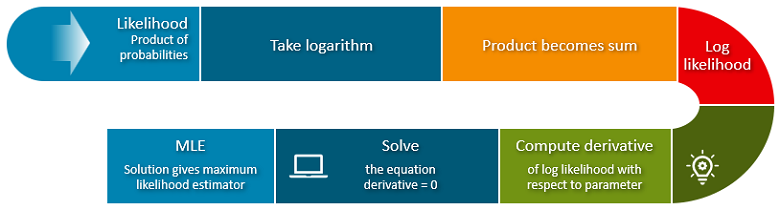## Assumptions

We observeindependent draws from a Poisson distribution.

In other words, there areindependent Poisson random variablesand we observe their realizationsThe probability mass function of a single drawis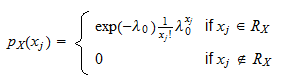where:

•is the parameter of interest (for which we want to derive the MLE);

• the support of the distribution is the set of non-negative integer numbers:•is the factorial of.

## The likelihood function

The likelihood function isProof

Theobservations are independent. As a consequence, the likelihood function is equal to the product of their probability mass functions:Furthermore, the observed valuesnecessarily belong to the support. So, we have## The log-likelihood function

The log-likelihood function is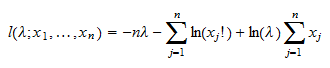Proof

By taking the natural logarithm of the likelihood function derived above, we get the log-likelihood: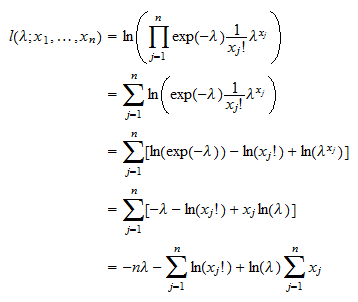## The maximum likelihood estimator

The maximum likelihood estimator ofisProof

The MLE is the solution of the following maximization problemThe first order condition for a maximum isThe first derivative of the log-likelihood with respect to the parameteris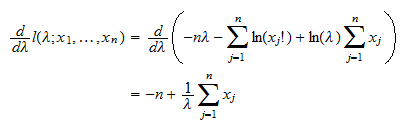Impose that the first derivative be equal to zero, and getTherefore, the estimatoris just the sample mean of theobservations in the sample.

This makes intuitive sense because the expected value of a Poisson random variable is equal to its parameter, and the sample mean is an unbiased estimator of the expected value.

## Asymptotic variance

The estimatoris asymptotically normal with asymptotic mean equal toand asymptotic variance equal toProof

The score is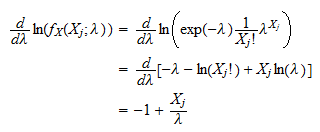The Hessian is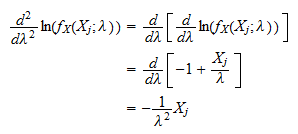The information equality implies that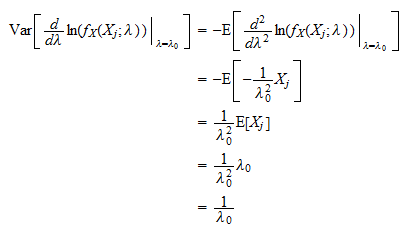where we have used the fact that the expected value of a Poisson random variable with parameteris equal to. Finally, the asymptotic variance is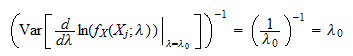Thus, the distribution of the maximum likelihood estimatorcan be approximated by a normal distribution with meanand variance.

## Other examples

On StatLect you can find detailed derivations of MLEs for numerous other distributions and statistical models.

TypeSolution
Exponential distributionUnivariate distributionAnalytical
Normal distributionUnivariate distributionAnalytical
T distributionUnivariate distributionNumerical
Multivariate normal distributionMultivariate distributionAnalytical
Normal linear regression modelRegression modelAnalytical
Logistic classification modelClassification modelNumerical
Probit classification modelClassification modelNumerical
Gaussian mixtureMixture of distributionsNumerical (EM)